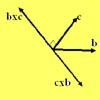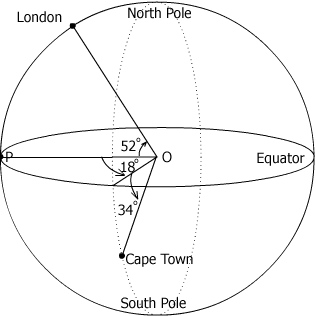#### You may also likeA quadrilateral changes shape with the edge lengths constant. Show the scalar product of the diagonals is constant. If the diagonals are perpendicular in one position are they always perpendicular?As a quadrilateral Q is deformed (keeping the edge lengths constnt) the diagonals and the angle X between them change. Prove that the area of Q is proportional to tanX.### Multiplication of Vectors

An account of multiplication of vectors, both scalar products and vector products.

# Air Routes

##### Age 16 to 18Challenge LevelLondon is situated at longitude $0^o$, latitude $52^o$ North and Cape Town at longitude $18^o$ East, latitude $34^o$ South. Taking the earth to be a sphere with unit radius (and ultimately scaling by 6367 kilometres for the radius of the earth) work out coordinates for both places, then find the angle LOC where L represents London, O the centre of the earth and C Cape Town. Hence find the distance on the surface of the earth between the two places. If a plane flies at an altitude of 6 kilometres and the journey takes 11 hours what is the average speed?

[You might also like to try the problems 'Over The Pole', which is a little simpler, and 'Flight Path' which is similar to 'Air Routes' but the method of solution given there is a bit different. ]